If you are starting to learn Common Lisp, and have already read the chapter about “Cons” from an introductory book (also called cons cells), then you could try the following exercise. How to represent a tree using nothing but conses; and abstract that implementation with functions to create and traverse the tree.

PLEASE NOTE, that if you need to represent trees in a production program you shouldn’t use lists as described here unless you have a good reason. This is only an exercise in understanding how cons cells work.

## 1. A Tree

Suppose we would like to represent the following tree in memory, using only lists (which are created by chaining conses). You should already know how a cons is created and what parts constitute one. If not, go back to an introductory book on Common Lisp.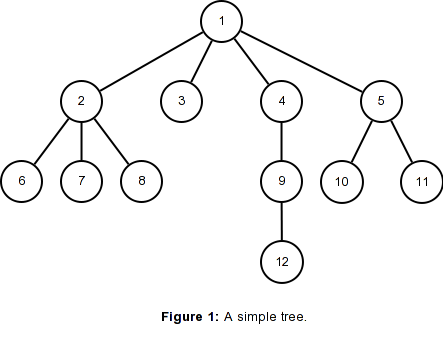This tree can be represented as a set of linked lists, like in the following diagram: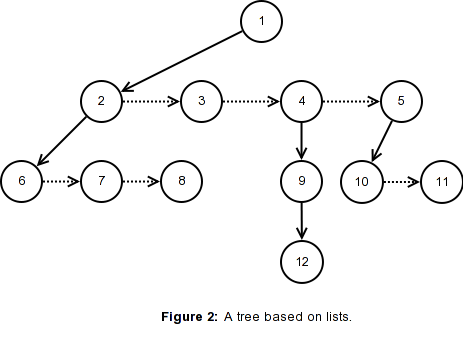The natural way to represent the previous tree as a Common Lisp list is like the following:

``(1 (2 6 7 8) 3 (4 (9 12)) (5 10 11))``

The first element of a list is the root of that tree (or a node) and the rest of the elements are the subtrees (more nodes). For example, the subtree `(2 6 7 8)` is a tree with “2” at its root and the elements “6 7 8” as its children.

### Exercise

How would you draw a diagram of the cons cells of the previous list? Compare it to the figure below.

.

.

.

.

.

.

.

.

.

.

The cons cells that build up the list above can be drawn like this: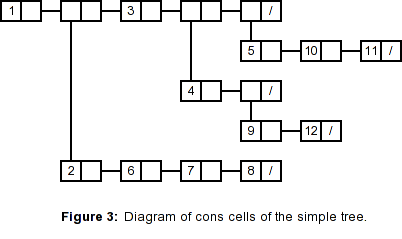## 2. Another representation of the Tree

The list representing the tree shown above is intuitive and easy to understand, however there’s a slight inconvenience. A subtree can be represented as a cons that directly contains the data in it’s car position, like in the case of “3”, or as a cons that refers to another cons that starts a proper list, like in the case of `(2 6 7 8)`.

There’s no consistency, when you need to access the data contained in a node, you must first check if the car position of the cons refers to a list, in which case you have to access that list to get the data.

Also, in the example list above, we are storing numbers in the nodes of the tree. What if you wanted to reference any kind of data from a node?, including a list?

### Exercise

How would you represent a single node of a tree, so that the methods to access the data, the children, and the next sibling are always the same?, how could the node reference any kind of data, including other lists?

Compare it to the representation given below.

.

.

.

.

.

.

.

.

.

.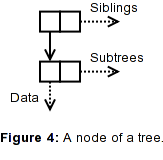There could be more than one way to represent a node, but in the rest of this small article we are going to represent them like in the figure above.

A tree node consists of two conses, the cdr of the first cons refers to the siblings of the node, while the cdr of the second cons refers to the subtrees (children) of the node. The car of the first cons refers to the second cons, while the car of the second cons refers to the data stored in this node, which can be any kind of object.

Using this node representation, the example tree given in Figure 1 would be displayed by Common Lisp as the following list. Can you see why?

``((1 (2 (6) (7) (8)) (3) (4 (9 (12))) (5 (10) (11))))``

### Exercise

How would you draw a diagram of the cons cells of the previous list? Compare it to the figure below.

.

.

.

.

.

.

.

.

.

.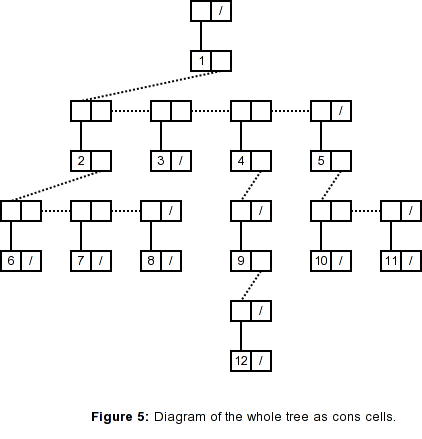## 3. The API

These are the functions that we are going to define for creating and traversing the nodes of a tree.

``````(defun make-tree (data)
"Creates a new node that contains 'data' as its data."
...)

"Takes two nodes created with 'make-tree' and adds the
second node as a child of the first. Returns the first node,
which will be modified."
...)

(defun first-child (tree)
"Returns a reference to the first child of the node passed in,
or nil if this node does not have children."
...)

(defun next-sibling (tree)
"Returns a reference to the next sibling of the node passed in,
or nil if this node does not have any siblings."
...)

(defun data (tree)
"Returns the information contained in this node."
...)``````

Notice that the function `add-child` will modify the node passed as the first argument, it is a destructive function, it has side effects.

### Exercise

Given the structure of a node as shown in Figure 4 above, come up with the code for the functions outlined above.

Compare your code to the solutions given below.

Test your code with the following usage example:

``````(let ((one (make-tree 1)))
(let ((two (first-child one)))
(let ((four (next-sibling two)))
one)

=> ((1 (2 (6) (7)) (4 (9 (12))) (5)))``````

.

.

.

### Code for the functions outlined above

I’m going to skip showing a code for the function `add-child` for now, we are going to discuss it in the next section.

The first function `make-tree` just needs to create the two conses as depicted in Figure 4, and place the data in the correct place.

``````(defun make-tree (data)
"Creates a new node that contains 'data' as its data."
(cons (cons data nil) nil))``````

Similarly, for the other functions, we just need to follow the correct cells as suggested in Figure 4.

``````(defun first-child (tree)
"Returns a reference to the first child of the node passed in,
or nil if this node does not have children."
(cdr (car tree)))

(defun next-sibling (tree)
"Returns a reference to the next sibling of the node passed in,
or nil if this node does not have any siblings."
(cdr tree))

(defun data (tree)
"Returns the information contained in this node."
(car (car tree)))``````

Easy, is it not?. Were you checking for null trees? That is, where you doing something like this?

``````(defun first-child (tree)
(if (null tree)
nil
(cdr (car tree))))``````

or

``````(defun first-child (tree)
(if (null (cdr (car tree)))
nil
(cdr (car tree))))``````

Well, don’t, that’s Java/C++ thinking. Notice that `(car nil)` evaluates to `nil`, and similarly, `(cdr nil)` evaluates to `nil` too.

However, trying to pass an atom or other object that is not a cons to either car or cdr is an error. For example `(first-child 3)` would cause an error.

You could try to guard against that redefining `first-child` like this:

``````(defun first-child (tree)
(when (listp tree)
(cdr (car tree))))``````

However, I wouldn’t do that, because I think that signalling an error on a function call like `(first-child 3)` is the correct thing to do. The user of the function is the one that is committing an error, not the implementor of the function.

## 4. First approach to add-child

There are a few ways you could have implemented the function `add-child`, let’s consider an example that at first sight might look fine.

``````(defun add-child (tree child)
(setf (car tree) (append (car tree) child))
tree)``````

Remember that the cdr of the second cons `(car tree)` refers to the children of the node, so that when we do `(append (car tree) child)` we are appending `child` to the end of the list of conses that start with the one in `(car tree)`. Since append does not modify any of its arguments and instead returns a fresh “consed” list (a new copy), we have to capture it again with `(setf (car tree) ...)`.

By the way, the setf line can be replaced with this line,

``(rplaca tree (append (car tree) child))``

rplaca stands for “replace car”, and it replaces the car of the first argument with whatever the second argument evaluates to.

Now, let’s try building the whole tree depicted in Figure 1; the following code can accomplish that.

``````(let ((one (make-tree 1)))
(let ((two (first-child one)))
(let ((four (next-sibling (next-sibling (first-child one)))))
(let ((five (next-sibling four)))
one)

=> ((1 (2 (6) (7) (8)) (3) (4 (9 (12))) (5 (10) (11))))``````

By visually inspecting the resulting list you can see that the tree is being built correctly. But you might wonder why juggle with `first-child` and `next-sibling` with nested let bindings, why not create the nodes that we need to refer more than once in the first let binding.

``````(let ((one    (make-tree 1))
(two    (make-tree 2))
(four   (make-tree 4))
(five   (make-tree 5)))
one)

=> ((1 (2) (3) (4) (5 (10) (11))))``````

What??, the children of nodes “2” and “4” were lost, but somehow the children of node “5” were added correctly. There’s no difference in the way we create nodes 2, 4 and 5, and there’s no difference in how we add children to them either. What’s going on?

### Exercise

This is where you will really test your understanding of how conses work and how references to objects in Common Lisp work. Try to figure out why the function `add-child` as used above is failing to correctly build the tree that we want.

Drawing the cons cells after each operation will be helpful.

You will also need to be sure to understand how append works.

.

.

.

.

.

.

.

.

.

.

## 5. Understanding what is going wrong

To understand what is going wrong, let’s see what happens after let creates the bindings, but before any form inside the let body is evaluated.We have four variables bound to four tree nodes. Then after evaluating the form `(add-child one two)` we have the following objects.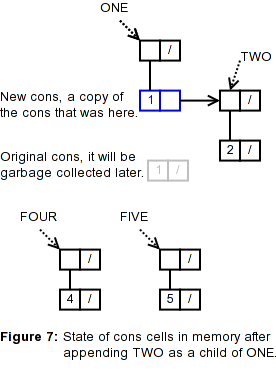The function append does not modify any of its arguments, and therefore to be able to build a new list it has to create new conses copying the top list structure of all the lists it has been passed in as arguments, except for the very last list, which will be just pointed to by the second to last list.

After `(append (car tree) child)` has created the new list, we point to it by doing `(setf (car tree) ...)`, thereby losing the reference to the original cons that was there (shown in grey in the above figure).

Please Note, the figure above may create a confusion. I’ve been drawing the numbers inside the cons for simplicity, but this is not very accurate. The two components of a cons (the car and the cdr) can refer to any type of object, and these objects may live in other parts of the memory.

Sometimes an implementation may store integers and other data types directly inside the cons, but you shouldn’t care or depend on this.

Functions like append only copy the “list structure”, it will never copy the data that a cons refers to. (But make sure to understand the difference between append and copy-tree, refer to your Common Lisp book or the HyperSpec.)

A more accurate depiction of the cons cells and the objects they refer to would look like this.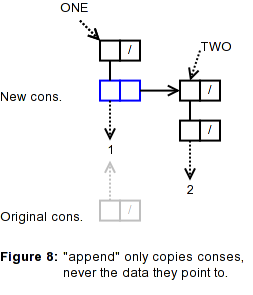However, I’ll continue drawing the numbers inside the cons for simplicity.

OK, so moving on, after the form `(add-child one (make-tree 3))` has been evaluated, we have the following situation.append copied the top list structure of the first list it had as an argument, this new list points to the last list, the node “3”. “Top list structure” means that append will only copy the conses that it reaches by following the cdrs of each cons; it will never go down the car of any cons (that’s what copy-tree would do).

The conses of the new list are shown in blue, while the original cons that was holding the value “1” and pointing to TWO is shown in grey. Since nothing is pointing to this grey cons, it may be garbage collected.

Now let’s see what happens after evaluating `(add-child one four)`.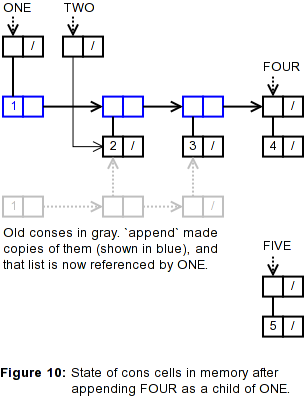Again, we see the new copies of conses marked in blue, while the original conses are marked in grey. Since those grey conses can’t be reached anymore, they may eventually be garbage collected.

And, after evaluating `(add-child one five)` we have the following.Again, the blue conses are the copies made by append and the grey conses here were the blue conses of Figure 10. Can you see why there’s only three grey conses (which can be GC) and four new blue conses?

So far everything seems to be working ok. But now comes the interesting part.

### Exercise

From the state of cons cells depicted in Figure 11 above, can you see what will happen after the form `(add-child two (make-tree 6))` is evaluated?, can you see where lies the problem?. Drawing the conses could be helpful.

Compare it to the figure below.

.

.

.

.

.

.

.

.

.

.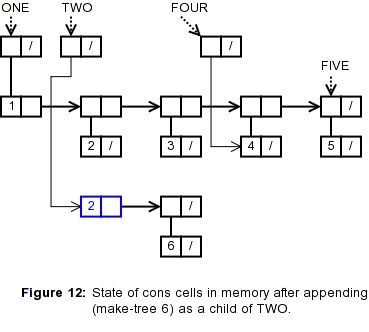Let’s recall the definition of `add-child`:

``````(defun add-child (tree child)
(setf (car tree) (append (car tree) child))
tree)``````

We can see that we are calling append on `(car tree)` and `child`, which correspond to `(car TWO)` and the value of `(make-tree 6)`. Therefore it’s easy to see that append is creating a copy of the cons in `(car TWO)`, returning a new list. Finally, setf sets the `(car TWO)` to point to this new list, completely separating `TWO` from the original tree starting with `ONE`.

Now we have two disjoint trees, one starting at the node pointed to by `ONE` and the other starting at the node pointed to by `TWO`. If we keep adding children to `TWO`, they will not be reachable from `ONE`. Likewise if we add children to the node `FOUR`.

Can you see why there’s no problem in adding children to the node `FIVE`?

## 6. A correct version of add-child

The problem with the definition of `add-child` above is that while it is defined to modify the argument `tree`, it’s actually only modifying part of it, and making new copies of other parts of `tree` (its children).

In other words, we should consider that the argument `tree` refers not only to a single node, but to a whole “tree” with subtrees. Of course the fact that we named the argument `tree` is to remind us of that.

We have to be always careful when designing our functions, and be clear if they will modify their arguments and how; or otherwise specify that our function will be completely side-effect free.

When we say that the function `add-child` will modify the first argument to add the second argument as a child of it, then it must do that, but do it to the whole conceptual tree.

The other alternative is to build a side-effect free `add-child`, which will create a new copy of the whole tree with the child appended, and leave the responsibility of capturing that new tree and updating any and all references to it or parts of it, to the user of our function.

So how do we correct `add-child` to do the correct thing?. We know that we can’t use append because it creates new copies of the children that `tree` had. Knowing that, the solution is easy, we just need to use the destructive version of append, which is called nconc.

nconc won’t create any copy of anything, and instead will modify it’s arguments so that they are linked in a single list, much like what append does.

``````(defun add-child (tree child)
"Takes two nodes created with 'make-tree' and adds the
second node as a child of the first. Returns the first node,
which will be modified."
(nconc (car tree) child)
tree)``````

nconc will modify the cdr of the second cons of `tree` to add the `child` if it had not previous children; otherwise it will modify the last node in the list of children of `tree`, and link the `child` to it.

Can you see why it’s just `(nconc (car tree) child)` and there’s no need to use setf?

But be very careful, nconc is the only destructive function for which you don’t need to capture it’s result with setf. When you are using other destructive functions like nreverse you must always capture the result with setf.

## 7. Traversing the tree

Finally, it’s difficult to see the returned list and interpret it. Let’s try to create a function to traverse the tree as the last exercise.

``````(let ((one    (make-tree 1))
(two    (make-tree 2))
(four   (make-tree 4))
(five   (make-tree 5)))

;; Print the contents of the tree,
(traverse one)
;; and return it.
one)``````

If we input the above into the REPL, we should get the following output.

``````Data: 1  Children: (2 3 4 5)
Data: 2  Children: (6 7 8)
Data: 6
Data: 7
Data: 8
Data: 3
Data: 4  Children: (9)
Data: 9  Children: (12)
Data: 12
Data: 5  Children: (10 11)
Data: 10
Data: 11
((1 (2 (6) (7) (8)) (3) (4 (9 (12))) (5 (10) (11))))``````

Notice that the list `((1 (2 ...)` is the value of `one` returned by the let form, while the information before it is being printed by `(traverse one)`.

### Exercise

Write the function `traverse` so that it generates the output shown above. Compare it to the solution given below.

Tip: you can use the `"~v@T"` directive to the format function to move the cursor forward. For example:

``````(format t "~v@TFoo" 2)
=>  Foo

(format t "~v@TBar" 4)
=>    Bar

(format t "~v@TFoo" 6)
=>      Foo``````

.

.

.

.

.

.

.

.

.

.

Let’s build the solution in steps. First we should print the node data:

``(format t "~&~v@TData: ~A" padding (data tree))``

The parameter `padding` will tell format to indent the line a certain number of columns. We’ll see how to calculate that later. The `"~&"` directive tells format to print a newline only at the beginning of a line.

We also need to print the children of the node, on the same line, but only if there are children to print.

``````(format t "~&~v@TData: ~A" padding (data tree))
(when (first-child tree)
(format t "  Children: ~A" (first-child tree)))``````

But wait, that’s not correct, it would print the whole tree of the children of node, like this:

``Data: 1  Children: ((2 (6) (7) (8)) (3) (4 (9 (12))) (5 (10) (11)))``

We only want the data in the nodes of the direct children of the node, as a list. We can use the maplist function for that. maplist applies a function to successive sublists of a list.

``````(format t "~&~v@TData: ~A" padding (data tree))
(when (first-child tree)
(format t "  Children: ~A"
(maplist #'(lambda (x) (data x))
(first-child tree))))``````

Now let’s wrap this in a function.

``````(defun traverse (tree &optional (padding 0))
(format t "~&~v@TData: ~A" padding (data tree))
(when (first-child tree)
(format t "  Children: ~A"
(maplist #'(lambda (x) (data x))
(first-child tree)))))``````

OK, now that we have printed the data of the node and a list of the children, we have to repeat this process for each of the children of this node, and they should be printed indented below the current node.

Since we already have the code to print the node and a list of its children, we will reuse this function and do a recursive call. One important thing to keep in mind when writing a recursive function is write a test for ending the recursion. In this case the recursion must end when the parameter `tree` is `nil`.

``````(defun traverse (tree &optional (padding 0))
(when tree
(format t "~&~v@TData: ~A" padding (data tree))
(when (first-child tree)
(format t "  Children: ~A"
(maplist #'(lambda (x) (data x))
(first-child tree))))
(traverse (first-child tree) (+ padding 3))))``````

We can see above how the `padding` is being incremented, in this case by 3 columns. Since the parameter `padding` is optional, in the first invocation `(traverse one)` the `padding` will be zero, and then it will be incremented by 3 on each recursive invocation.

But wait, the above code will only print the leftmost nodes in the tree, we still need to print the siblings of the nodes. That is very easy.

``````(defun traverse (tree &optional (padding 0))
(when tree
(format t "~&~v@TData: ~A" padding (data tree))
(when (first-child tree)
(format t "  Children: ~A"
(maplist #'(lambda (x) (data x))
(first-child tree))))
(traverse (first-child tree) (+ padding 3))
Since the siblings should be printed at the same level as the current node, the `padding` is left unmodified.